Home > A2C > Chapter 8 > Lesson 8.1.2 > Problem8-19

8-19.
1. Evaluate each expression without using a calculator or changing the form of the expression. Homework Help ✎1. log(1)

2. log(103)

3. 10log(4)

4. 103log(4)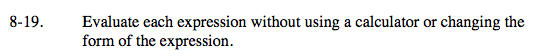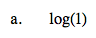What power would you raise 10 to to equal 1?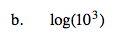Use the power property of logs:3 · log(10).
What is log(10)? What is 3 · log(10)?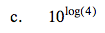Use the Inverse Relationship:

$\text{log}_m(m)^n\:\text{and}\:m^{\text{log}_m(n)} = n$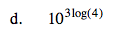Combine the strategies from parts (b) and (c).